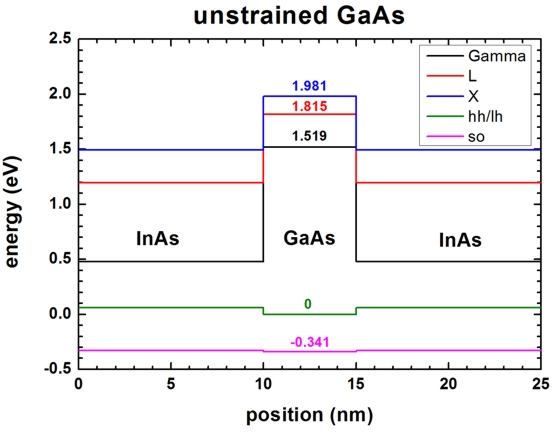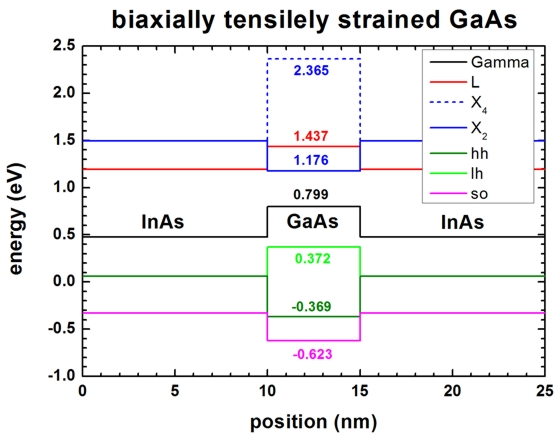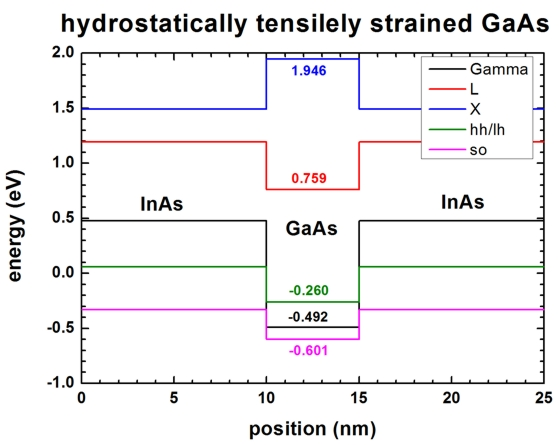nextnano.com  nextnano³  Download | Search | Copyright | Publications  * password protected nextnano³ software1D deformation potentials

# nextnano3 - Tutorial

## Strain: Band shifts and splittings due to conduction and valence band deformation potentials

Author: Stefan Birner

If you want to obtain the input files that are used within this tutorial, please check if you can find them in the installation directory.
If you cannot find them, please submit a Support Ticket.
```-> 1Ddeformation_potentials_no_strain_nn3.in                / *_nnp.in - ```input file for the nextnano3 and nextnano++ software``` -> 1Ddeformation_potentials_strain_nn3.in                   / *_nnp.in - ```input file for the nextnano3 and nextnano++ software``` -> 1Ddeformation_potentials_ReadInStrainTensor_hydro_nn3.in / *_nnp.in - ```input file for the nextnano3 and nextnano++ software``` -> 1Ddeformation_potentials_hydro.in                                   - ```input file for the nextnano3 software```  ```

## InAs/GaAs/InAs structure

• This input file simulates an InAs/GaAs/InAs structure.
The structure is grown pseudomorphically on InAs, i.e. the GaAs is tensilely strained, the InAs is unstrained. The growth direction  is along z, the interfaces are in the (x,y) plane.
• We artificially set the electrostatic potential to zero to avoid any further shifting of the band edges.

By default Varshni parameters are used to determine temperature dependent band gaps (i.e. temperature dependent` conduction-band-energies`).
Here, the Varshni parameters are switched off, thus the conduction and valence band edges from the database (or input file) are taken.
``` \$numeric-control  simulation-dimension            = 1  zero-potential                  = yes  varshni-parameters-on           = no ! ``` Band gaps independent of temperature. Absolute values from database are taken.
``` lattice-constants-temp-coeff-on = no ! ```Lattice constants independent of temperature. Absolute values from database are taken.
`\$end_numeric-control`

### No strain

`-> 1Ddeformation_potentials_no_strain.in`

• The following figure shows the conduction and valence band edges of the heterostructure when no strain is applied (```strain-calculation = no-strain```). The heavy and light hole bands are degenerate.• GaAs:
``` conduction-band-energies = 2.979d0 3.275d0 3.441d0 ! [eV] Gamma, L, X```
``` valence-band-energies    = 1.346d0                 ! [eV] Ev,av  6x6kp-parameters         = ...     ...     ...     !                             0.341d0                 ! [eV] ```Deltasplit-off``` ```Conduction bands:
``` -> Gamma          band   = 2.979  -> L              band   = 3.275  -> X              band   = 3.441 ```Valence bands:
``` -> heavy     hole band   = 1.346 + 1/3 * 0.341 = 1.45967   (= Ev,av + 1/3 * ```Deltasplit-off``` = Ev,max)  -> light     hole band   = 1.346 + 1/3 * 0.341 = 1.45967   (= Ev,av + 1/3 * ```Deltasplit-off``` = Ev,max)  -> split-off hole band   = 1.346 - 2/3 * 0.341 = 1.11867   (= Ev,av - 2/3 * ```Deltasplit-off``` = Ev,max - ```Deltasplit-off```) ```Note: We apply an overall band-shift to all bands in order to align the topmost valence bands (heavy hole/light hole) to zero (0 eV).```  !-----------------------------------------------  ! Shift all bands, so that GaAs (hh/lh) = 0 eV.   ==>   Ev,max = 0  !-----------------------------------------------  band-shift = -1.45967d0 ! [eV] ```Conduction bands:
``` -> Gamma          band   = 2.979   - 1.45967 =  1.519  -> L              band   = 3.275   - 1.45967 =  1.815  -> X              band   = 3.441   - 1.45967 =  1.981 ```Valence bands:
``` -> heavy     hole band   = 1.45967 - 1.45967 =  0         (= Ev,max)  -> light     hole band   = 1.45967 - 1.45967 =  0         (= Ev,max)  -> split-off hole band   = 1.11867 - 1.45967 = -0.341 ```For details of the calculations of the band gap or band edges see FAQ section.

### Strain (biaxial strain)

`-> 1Ddeformation_potentials_strain.in`

• Then we turn on strain (```strain-calculation = homogeneous-strain```). Here it is interesting to see that the six-fold degenerate X bands in GaAs split.• Valence bands``` absolute-deformation-potential-vb   = -1.21d0                 ! av  [eV] ```(Ref. Zunger)
```uniax-vb-deformation-potentials     = -2.0d0  ...             ! b,d [eV]```
InAs has a larger lattice constant than GaAs. The tensile strain for GaAs has the following three components (the offdiagonal strain components are zero):
```e||  = exx = eyy = 0.07165 e_|_ = ezz = - 0.06643 ```The hydrostatic strain is the trace of the strain tensor and corresponds to the change in volume dV/V:``` ehydro = Tr(eij) = exx + eyy + ezz = 2e|| + e_|_ = 0.07687 ```
The three valence bands shift by a constant amount (hydrostatic or absolute deformation potential):
```Ev,av' = Ev,av + av ehydro = Ev,av + (-1.21 ehydro) = Ev,av - 0.0930127```

In addition to this shift each hole band gets a further shift which depends on the growth direction: For growth direction along  the additional valence bands shift in the following way:
```Ev*(hh) = - 1/2 Esh001 Ev*(lh) = - 1/2 Delta``````so + 1/4 Esh001 + 1/2 [ SQRT(Deltaso2 + Deltaso Esh001 + 9/4 (Esh001)2 ) ] Ev*(so) = - 1/2 Deltaso + 1/4 Esh001 - 1/2 [ SQRT(Deltaso2 + Deltaso Esh001 + 9/4 (Esh001)2 ) ] ```These three equations include the spin-orbit split-off energy``` Deltaso ```and are thus given relative to the unstrained valence band edge maximum``` Ev,max = Ev(hh) = Ev(lh)```.``` ```Note that without strain, these equations lead to:``` Ev*(hh) = 0 Ev*(lh) = 0 Ev*(so) = - Deltaso ```
```Esh001 = -b(exx + eyy - 2ezz) = 2b(e_|_-e||) = 2(-2.0)(e_|_-e||) = 0.55232```

```Ev(hh) = Ev,max + av ehydro + Ev*(hh) =  Ev,max - 0.0930127 + ( - 0.27616 )                     = -0.369 Ev(lh) = Ev,max + av ehydro + Ev*(lh) =  Ev,max - 0.0930127 + ( - 0.1705 + 0.13808 + 0.497745 ) =  0.372 Ev(so) = Ev,max + av ehydro + Ev*(so) =  Ev,max - 0.0930127 + ( - 0.1705 + 0.13808 - 0.497745 ) = -0.623 ```In our example, we have shifted the bands (`band-shift = -1.45967d0`), so that it holds for unstrained GaAs:``` Ev,max = 0  ```
• Conduction bands``` absolute-deformation-potentials-cbs = -9.36d0 -4.91d0 -0.16d0 ! ac(Gamma), ac(L), ac(X) uniax-cb-deformation-potentials     =  0d0    14.26d0  8.61d0 ! Xi(Gamma), Xi(L), Xi(X) ```Each of the three conduction bands shifts by a constant amount (hydrostatic or absolute deformation potential):
```Ec'(Gamma) = Ec0(Gamma) + ac(Gamma) ehydro = Ec0(G) + (-9.36 ehydro) = 1.519 - 0.7195 = 0.799```
```Ec'(L)     = Ec0(L)     + ac(L)     ehydro = Ec0(L) + (-4.91 ehydro) = 1.815 - 0.3774 = 1.437```
```Ec'(X)     = Ec0(X)     + ac(X)     ehydro = Ec0(X) + (-0.16 ehydro) = 1.981 - 0.0123 = 1.9687```

The X conduction band of GaAs is also subjected to a splitting (upwards and downwards relative to `Ec'(X)`). This amount depends on the growth direction. For  growth direction the splitting is given by
```Ec001*(X)    =   2/3 Xi(X) (e_|_-e||) =   2/3 * 8.61 * (-0.13808) = - 0.7925792```
```Ec100,010*(X) = - 1/3 Xi(X) (e_|_-e||) = - 1/3 * 8.61 * (-0.13808) =   0.3962896```

```--> Ec001(X)    = Ec'(X) + Ec001*    (X) = 1.9687 - 0.7925792 = 1.176 ```(2-fold valley degeneracy)``` --> Ec100,010(X) = Ec'(X) + Ec100,010*(X) = 1.9687 + 0.3962896 = 2.365 ``` (4-fold valley degeneracy)
• The above given equations for the valence band are not used inside nextnano³, however. We get the band shifts by diagonalizing the Bir-Pikus strain Hamiltonian which is given in Basics 2 (strain effects). This is a more general approach as it gives the correct shifts for arbitrary orientations (However, it is only for valence bands). The equations above are the special case for growth along the  direction.
• Note: There are two different definitions of the valence band deformation potential in the literature. What can be measured experimentally is the deformation potential of the band gap which is composed of the deformation potential of the valence and conduction band edge.
```agap = ac + av     ```(e.g. definition in Vurgaftman1)
One can also argue that the conduction band deformation potential can be constructed from the valence band edge and the band gap deformation potential like this:
```ac = av + agap     ```(definition in nextnano³)
The equations differ in the sign of the valence band deformation potential. Note that the Erratum of Bahder1 is not in agreement with the definiton inside nextnano³.

Note also the comment of Vurgaftman1: "... the band gap increases for a compressive strain. Under positive hydrostatic pressure, i.e. negative strain, the change in energy``` DeltaEgap = agap(exx + eyy + ezz) ```must be positive. This implies a negative value for` agap`. [...] It is generally believed that the conduction band edge moves upward in energy while the valence band moves downward, with most of the change being in the conduction band egde, although Wei and Zunger recently argued that this is not always the case."

Note also the comment of Wei and Zunger (Wei1): "We show that the volume deformation potentials ac of the conduction-band minimum state Gamma6c are usually large and always negative (energy increases with pressure) while the volume deformation potentials av of the VBM state Gamma8v are usually small and negative for zinc blende compounds containing occupied valence d state (e.g. GaAs, InAs) but positive for compounds without occupied valence d orbitals (e.g. AlAs)."

### Hydrostatic strain

```-> 1Ddeformation_potentials_ReadInStrainTensor_hydro_nn3.in / nnp*.in -> 1Ddeformation_potentials_hydro.in```

• Now we want to apply a hydrostatic strain to our 1D structure (```strain-calculation = hydrostatic-strain```). The difference to pseudomorphic strain is simply that the pressure acts equally from each direction. This leads per definition to:
```exx = eyy = ezz ```for cubic crystals.``` ```Normally` ezz `is calculated in the following way:``` ezz = - 2 (c12/c11) e````xx `(only for  growth direction!)``` ```For option``` hydrostatic-strain ```we artificially use inside the code the constant``` c12 = -c11/2 ```leading to `ezz=e``xx`.
This allows us to study the effects of absolute deformation potentials only (without splitting of the bands and without shifts due to uniaxial deformation potentials).
In this case we have:
```exx = eyy = ezz = 0.07165 ehydro = Tr(eij) = exx + eyy + ezz = 3exx = 0.21495 Ev' = Ev0 + av ehydro = Ev0 + (-1.21*0.21495) = Ev0 - 0.26009  ``````Ev(hh) = Ev0(hh) + av ehydro =  0     - 0.26009 = -0.260 Ev(lh) = Ev0(lh) + av ehydro =  0     - 0.26009 = -0.260 Ev(so) = Ev0(so) + av ehydro = -0.341 - 0.26009 = -0.601 Ec'(Gamma) = Ec0(Gamma) + ac(Gamma) ehydro = Ec0(G) + (-9.36 ehydro) = 1.519 - 2.0119 = -0.492```
```Ec'(L)     = Ec0(L)     + ac(L)     ehydro = Ec0(L) + (-4.91 ehydro) = 1.815 - 1.0554 =  0.759```
```Ec'(X)     = Ec0(X)     + ac(X)     ehydro = Ec0(X) + (-0.16 ehydro) = 1.981 - 0.0343 =  1.946```

Here we have negative hydrostatic pressure = increase in volume = positive hydrostatic strain.
The valence bands shift downwards (`av `is negative) and the conduction bands shift downwards, too (`ac `is negative).
In this case, the Gamma conduction band is below the heavy/light hole band edge.

Importing a strain tensor from a file

It is also possible to import a strain tensor from a file.
Here, we read in the file:``` StrainTensor_hydrostatic.dat ```In this example, we apply no strain to InAs and a hydrostatic strain to GaAs.

```exx = eyy = ezz = 0.07165 ehydro = Tr(eij) = exx + eyy + ezz = 3exx = 0.21495 ```
```position[nm]       eps_xx            eps_yy            eps_zz            eps_xy           eps_xz           eps_yz   9.500000000E+000  0.000000000E+000  0.000000000E+000  0.000000000E+000 0.000000000E+000 0.000000000E+000 0.000000000E+000 ! InAs  10.500000000E+000 71.649051431E-003 71.649051431E-003 71.649051431E-003 0.000000000E+000 0.000000000E+000 0.000000000E+000 ! GaAs ```

``` \$simulation-flow-control   flow-scheme        = 0                                           ! ```Here, we use``` flow-scheme = 0 ```as we only calculate the strain.```   strain-calculation = import-strain-simulation-coordinate-system  ! ```We import a strain tensor that is defined with respect to the simulation coordinate system from a file.```  \$end_simulation-flow-control  \$import-data-on-material-grid   source-directory   = "D:\My Strain Tensor folder\"   filename-strain    = StrainTensor_hydrostatic.dat  \$end_import-data-on-material-grid```